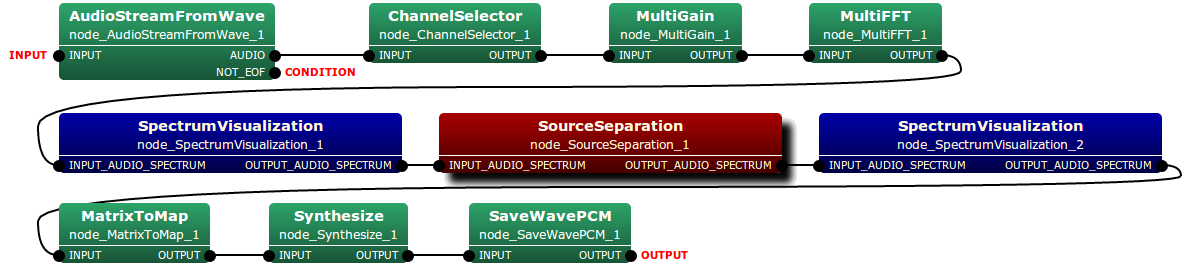# SourceSeparation Node¶

## Outline of the node¶

This node conducts blind sound source separation based on independent vector analysis.

## Typical connection¶

The type of both the input and output of SourceSeparation node is multi-channel (2-ch) audio spectrum. Typical connection of this node is depicted as follows:## Input-output and property of the node¶

### Input¶

INPUT_AUDIO_SPECTRUM Matrix<complex<float> >
Windowed spectrum data. A row index is channel, and a column index is frequency.

### Output¶

OUTPUT_AUDIO_SPECTRUM Matrix<complex<float> >
Windowed and speech-enhanced spectrum data . A row index is channel, and a column index is frequency.

### Parameters¶

Parameters of this node are listed as follows:

Parameter name Type Default value Unit Description
FFT_LENGTH int 512 sample Analysis frame length.
ITERATION_METHOD string FastIVA   Iteration method.
MAX_ITERATION int 700   Processing limitation: maximum number of iterations.
NUMBER_OF_SOURCE_TO_BE_SEPARATED int 2   Number of sound sources to be separated.
SEPARATION_TIME_LENGTH float 5.0 second Separation window length.
ADVANCE int 160 sample The length in sample between a frame and a previous frame.
SAMPLING_RATE int 16000 Hz Sampling rate.

## Details of the node¶

This module conducts recovery of the original sound signals from the combined sound signal by using independent vector analysis (IVA)  or Fast independent vector analysis (Fast-IVA) . In the case of IVA, the objective function is Kullback-Leibler (KL) divergence:

$$C={\rm constant}- \sum^F_f {\rm log}\left|{\rm det} W_{mkf}\right| - \sum^M_m E\left[{\rm log}P \left( \hat{S}_1, \cdots ,\hat{S}_M \right)\right]$$

where $$\hat{S}_m (m = 1, \cdots, M)$$ and $$W_{mkf}$$ represent the input signal of m-th microphone and the separation matrix of IVA, respectively. The lerning algorithm of IVA is based on natural gradient-descent method:

$$W^{new}_{mkf}=W^{old}_{mkf} + \eta \sum^K_k \left( I_{mk} - E \left[ \frac{\hat{S}_{kf}}{\sqrt{\sum^F_f \left| \hat{S}_{kf} \right|^2}} \hat{S}_{kf}^{\ast} \right] \right) W^{old}_{mkf}$$

where $$\eta$$ is learning rate (set at 0.1)

In the case of Fast-IVA, following modified objective function based KL divergence on is used:

$$C=-\sum^M_m E\left[{\rm log}P \left( \hat{S}_1, \cdots ,\hat{S}_M \right)\right] - \sum^M_m \beta\left[W^T_{mkf}W^{new}_{mkf}-1\right]$$,

where $$\beta$$ is Langrangian multiplier. The learning algorithm, on the other hand, is based on newton method with fixed point iteration:

$$W^{new}_{mkf}= E\left[\frac{1}{\sqrt{\sum^F_f \left|\hat{S}_{kf}\right|^2}} - \frac{\hat{S}^2_{kf}}{\left( \sqrt{\sum^F_f \left|\hat{S}_{kf}\right|^2}\right) ^3}\right] W^{old}_{mkf} -E\left[\frac{\hat{S}_{kf}}{\sqrt{\sum^F_f \left|\hat{S}_{kf}\right|^2}} X_{kf}\right]$$